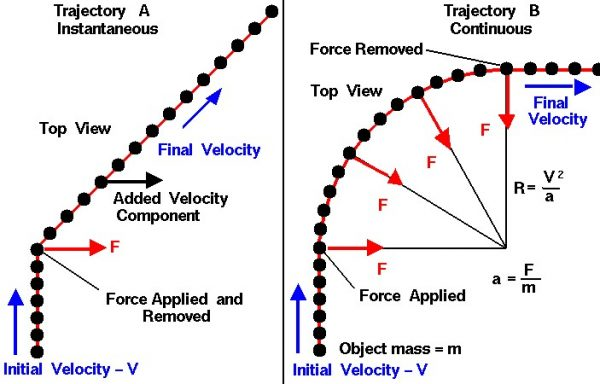# Object Motion Due to a Side Force## Force

Sir Isaac Newton first presented his three laws of motion in the “Principia Mathematica Philosophiae Naturalis” in 1686. His first law states that every object will remain at rest or in uniform motion in a straight line unless compelled to change its state by the action of an external force. When an external force F is applied, the object is accelerated a in the direction of the force with a magnitude directly proportional to the force and inversely proportional to the mass m of the object. This is a restatement of Newton’s second law of motion:

## Object

On this page we show two examples of the motion of an object which is subjected to a single external side force. In both cases, we are viewing the motion from above. An object, represented by a black circle, enters the domain from the bottom while moving at a constant initial velocity V. The small black circles represent the position of the object as time passes; the red line is the path, or trajectory, of the object’s motion. There is initially no external force acting on the object, so in keeping with Newton’s first law, the object moves upward at a constant velocity. The object is then subjected to a side force F.

## Trajectory A – Instantaneous Force

On the left side of the figure, the force F is directed to the right of the path and is instantaneously applied to the object. This type of force application occurs if the object experiences a collision with another object moving to the right. In response to Newton’s second law, the object receives an instantaneous acceleration to the right; the velocity to the right is changed from zero to some new value. Remember that forces, accelerations, and velocities are vector quantities having both a magnitude and a direction and that the acceleration of an object in response to an external force is in the direction of the force. Since the force is instantly removed, the object continues in a straight line motion as described by the first law. The object now has an additional component of velocity (to the right) which remains until some future external force acts on the object.

## Trajectory B – Continuous Force

On the right side of the figure, the force F is directed to the right of the path and is continuously applied to the object. This type of force application occurs if the object is subjected to an aerodynamic side force which is perpendicular to the flight direction, such as the banking turn of an aircraft, or a thrown, spinning ball. If the force remains constant, and is applied perpendicular to the motion, the resulting path is a circular arc. The radius of the circle is called the radius of curvature – R and it depends on the relative magnitude of the velocity V and the acceleration a generated by the side force.

### R = V ^2 / a

When the force is removed (banked aircraft brought to wings level) the object continues in a straight line motion as described by the first law. A continuous force applied perpendicular to the motion is called a centralized force since it produces a circular motion about some central point.

Provide feedback